# Nonparametric Bayes

## Concepts

Infinitively exchangeable
order of data does not matter for the joint distribution.

## Beta distribution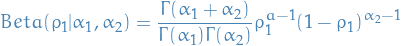1

### Overview

• Distribution over parameters for a binomial-distribution!
• So in a sense you're "drawing distributions"
• Like to think of it as simply putting some rv. parameters on the model itself, instead of simply going straight for estimating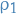in a binomial distribution.
• Remember the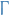function is a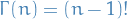when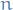is an integer.

## Dirichlet distribution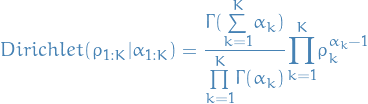2

### Overview

• Generialization of Beta distribution, i.e. over multiple categorical variables, i.e. distribution over parameters for a multionomial distribution.
• So if you say were to plot the Dirichlet distribution of some parameters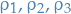we obtain the simplex/surface of allowed values for these parameters
• "Allowed" meaning that they satisfy being a probability within the multinomial model, i.e.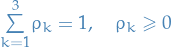• Got nice conjugacy properties, where it's conjugate to itself, and also multinomial distributions

### Generating Dirichlet from Beta

We can drawfrom a Beta by marginalizing over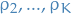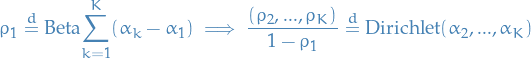3

This is what we call stick braking.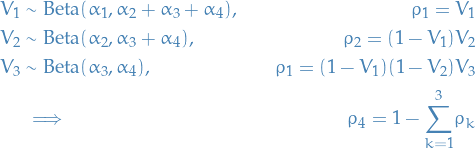4

## Dirichlet process

### Overview

• Taking the number of parameters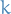to go to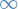.
• Allows arbitrary number of clusters =>can grow with the data

### Taking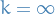We do what we do in Generating Dirichlet from Beta, the "stick braking". But in the Dirichlet process stick braking we do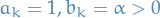5

And then we just continue doing this, drawing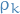as follows: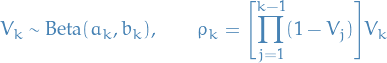6

Resulting distribution ofis then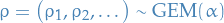where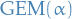is called the Griffiths-Engen-McCloskey (GEM) distribution.

To obtain a Dirichlet process we then do: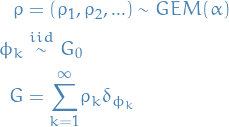7

where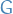can be any probability measure.

### Dirichlet process mixture model

Start out with Gaussian Mixture Model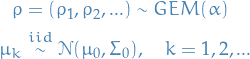8

Where our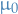and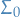are our priors of the Gaussian clusters. Which is the same as saying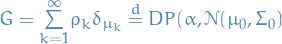.

So,is a sum over dirac deltas and so will only take non-zero values where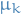corresponds to some. That is, it just indexes the probabilities somehow. Or rather, it describes the probability of each clusterbeing assigned to.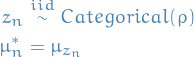9

i.e.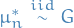, which means that drawing an assignment cluster for our nth data point, where the drawn cluster has mean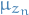, is equivalent of drawing the mean itself from.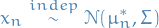i.e. the nth data point is then drawn from a normal distribution with the sampled mean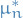and some variance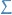.

The shape / variancecould also be dependent on the cluster if we wanted to make the model a bit more complex. Would just have to add some draw forin our model.

## Lecture 2

### Notation

•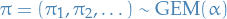which sums to 1 with probability one.
•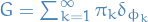•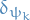is the dirac delta for the element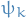### Stuff

•can be described as follows:
• Take a stick of length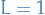•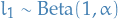• "Break" stick at the point corresponding to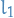:
•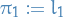•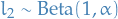• "Break" the rest of the stick by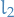:
•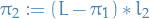•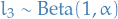• "Break" the rest of the stick:
•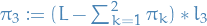• Then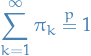• We let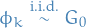where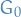is some underlying distribution

• The we define the random variable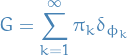whereis the dirac delta for the element• Thecan even be functions, ifis a distribution on a separable Banach space!
• Then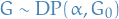where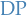denotes a Dirichlet process

• Observe thatdefines a measure!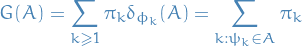hence ais basically a distribution over measures!

• So we have a random measure where the σ-algebra is defined by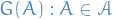where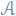is the original σ-algebra

There's a very interesting property of thedistribution.

Suppose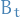is Brownian motion. Then consider the maximal points (i.e. new "highest" or "lowest" peak), then the time between these new peaks follow a!

We say a that a sequence of random variables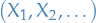is infinitely exchangable if and only if there exists an unique random measuresuch that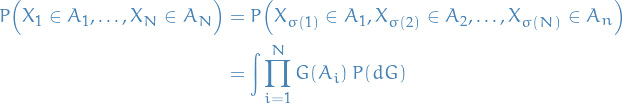Then observe that what's known as the Chinese restaurant process is just our previous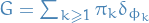where we've marginalized over all the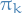!

### Dirichlet as a GEM

Suppose we have finite number of samples from a GEM distribution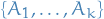.

Then,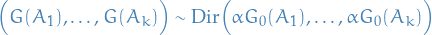Stochastic process on a σ-algebra.

A complete random measure is a random measure such that the draws are independent: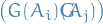## Appendix A: Vocabulary

categorical distribution
distribution with some probabilityfor the the class/label indexed by. So a multinomial distribution?
random measure